# PMean: How big is my graph?

When you are drawing a graph in R, sometimes you have to check how big things are before you can do anything else. There are many different ways of measuring size. Here are some simple examples. You can find the R Markdown code that created these examples here.

# plot size

When you are drawing a graph in R, sometimes you have to check how big things are before you can do anything else. There are many different ways of measuring size. Here are some simple examples.

``````# start without any extraneous variables
rm(list=ls())``````

## Set up a graph window

Anytime you draw a graph (for example, using the plot, barplot, boxplot, or hist command), you open up a window where that graph is displayed. The size of that window defaults to a particular value, but you can (and sometimes should) choose a different default for the size of your graph window. The windows command (or an equivalent command, x11, for all you Linux fans) allows you to open a window of a different size. For most of the examples shown here, you will see the values for the default window size.

Three warnings:

First, these are defaults on my system and your defaults may be different.

Second, I am running these graphs through knitr and R Markdown. If you run these commands directly from the command line, you will probably get different results than through knitr R markdown.

Third, R uses units of measurement like inches and centimeters that probably do not directly correspond to the physical size of the graph on your computer screen. In fact, if you are looking at this through a video projector, then the size of the screen and therefore the size of the graph window will change as you move the projector closer to or further away from the screen.

Size in inches and cenimeters still has value in a relative sense in that a graph windows is reported back as 4 inches will be half the size of something that is reported back as 8 inches and will be twice the size of something that is reported back as 2 inches.

## dev.size and fin

The dev.size() function produces the size of the entire graph window. This means the plotting region PLUS the marginal areas where the axes, the axis labels, and the title might be printed. Again, it uses a physical dimension of inches, which means something only in a relative sense.

``````ws.inches <- dev.size()
print(ws.inches)``````
``##  7 5``

Here I am showing the dev.size() without first opening a graphics window. In this case, the dev.size() function tells you the default size of a graphics window. This can vary from system to system and changes depending on where you open the graphics window from. Right now, my default graphics window is 7 inches in the horizontal dimension and 5 inches in the vertical dimension.

``````ws.fin <- par("fin")
print(ws.fin)``````
``##  6.999999 4.999999``

The par(“fin”) function does the same thing as dev.size() with one important exception (see below).

It is worth looking at the help file for par, because there are a lot of interesting things that par can help you with.

## size in pixels

You can change dev.size() to report in different units using the units argument (e.g., dev.size(unit=“cm”)) but centimeters are just like inches in that they represent a relative measure.

You can also report back the size of the entire graph window in pixels and pixels are not a relative measure.

``````ws.pixels <- dev.size(unit="px")
print(ws.pixels)``````
``##  1344  960``

The graphics window has 1344 pixels in the horixontal dimenion and `r ws.pixels` pixels in the vertical dimension.

``print(ws.pixels/ws.inches)``
``##  192 192``

This may differ on your system, but on my system each inch is considered to be equivalent to 192 pixels.

This can vary depending on your defaults and depending on where you open your graphics window from.

Pixels are a more precise way to represent the size of a graph and make more sense when you are moving from one display to another.

Even so, pixels are still ambiguous for some output options for graphs like pdf().

## Size of the plotting region

Once you set up a graph using plot or some other function, you create a plotting region and margins. The plotting region is where the lines, points, bars, etc. are drawn. The margins are where the axes, labels and titles are drawn.

Here are some ways of measuring the size of the plotting region.

``````x <- 1:19
y <- 10*c(1:10,9:1)
plot(x,y)``````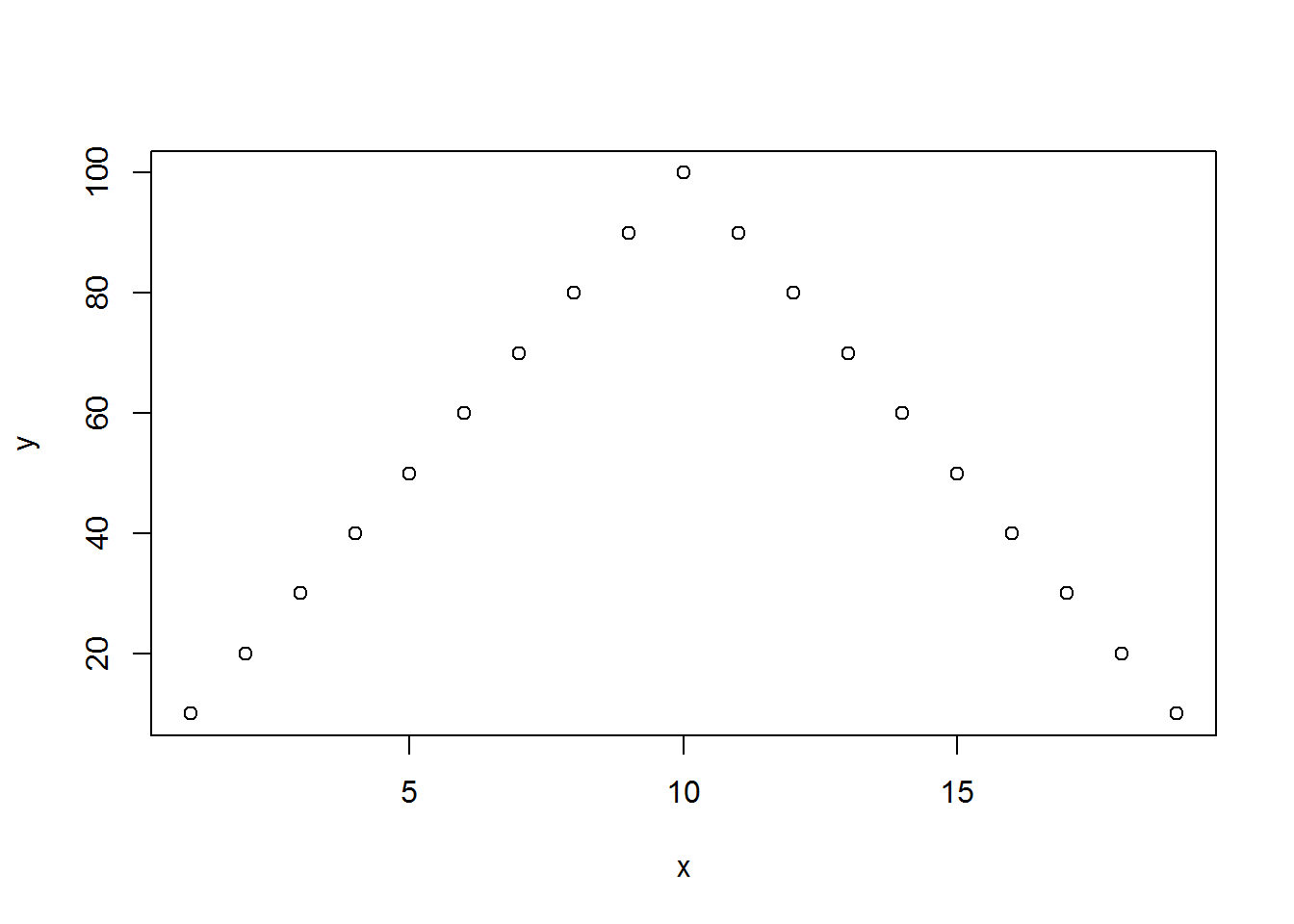``````gs.user <- par("usr")
print(gs.user)``````
``##    0.28  19.72   6.40 103.60``

The “usr” parameter shows the extremes in both the x and y dimensions in the user defined dimensions of the data. The minimum and maximum x values are 0.28 and 19.72 while the minimum and maximum y values are 6.4 and 103.6.

When R plots data, the default option is to add 4% to the end of either extreme so that points right on the edges don’t get clipped. The x values have a range of 18 (1 to 19) and 4% of 18 is 0.72. The y values have a range of 90 (10 to 100) and 4% of 90 is 3.6.

``````gs.prop <- par("plt")
print(gs.prop)``````
``##  0.1171429 0.9400000 0.2040000 0.8360000``

The “plt” parameter shows what proportion of the graphics window is taken up by the plotting region. You can control this several ways, and you might be tempted to make the plotting region equal to 100% of the graphics window. I’ve done this sometimes, but you should normally allow some room for other graphic elements like the axes and the title.

The horizontal (x) dimension of the graph starts 11.7% of the way from the left edge of the graphics window and ends 6% of the way from the right edge of the graphics window. The vertical (y) dimension of the graph starts 20.4% of the way from the bottom edge of the graphics window and ends 16.4% of the way from the top edge of the graphics window.

``````gs.inches <- par("pin")
print(gs.inches)``````
``##  5.759999 3.159999``

The “pin” parameter shows the size of the plotting region in inches. The x dimension is 5.76 inches, which is roughly you would get when you take away 11.7% and 6% from the 7 inches of width of the graphics window. Likewise the y dimension is 3.16 inches, which is what you would get when you take away 20.4% and 16.4% from the 5 inches of height of the graphics window.

# Comparing plot ranges

Once you are able to measure sizes in various dimensions, you can start to see how these all relate to one another.

``````user.range <- gs.user[c(2,4)]-gs.user[c(1,3)]
print(user.range)``````
``##  19.44 97.20``

The range in user coordinates is 19.4 and 97.2 units in the x and y axes, respectively.

``````prop.range <- gs.prop[c(2,4)]-gs.prop[c(1,3)]
print(prop.range)``````
``##  0.8228571 0.6320000``

The graph region takes up 82.3% and 63.2% of the space in the horizontal and vertical dimensions, respectively.

``````pixel.range <- ws.pixels*prop.range
print(pixel.range)``````
``##  1105.92  606.72``

Translated into pixels, the dimensions of the graphing region are 1106 by 607 pixels.

``````pixels.per.unit <- pixel.range / user.range
print(pixels.per.unit)``````
``##  56.888888  6.241975``

On the x-axis, each unit is equal to 57 pixels and on the y-axis, each unit is equal to 6 pixels.

It took a lot of tedious work to get to this point, but it is important sometimes to know the pixel to unit ratio. Suppose, for example, that you want to draw a circle with a diameter of 8 units in the x-direction. It would look funny if you assumed that the diameter in the y-direction was also 8 units.

``````radians <- seq(0,2*pi,length=30)
plot(x,y)
polygon(false.circle)``````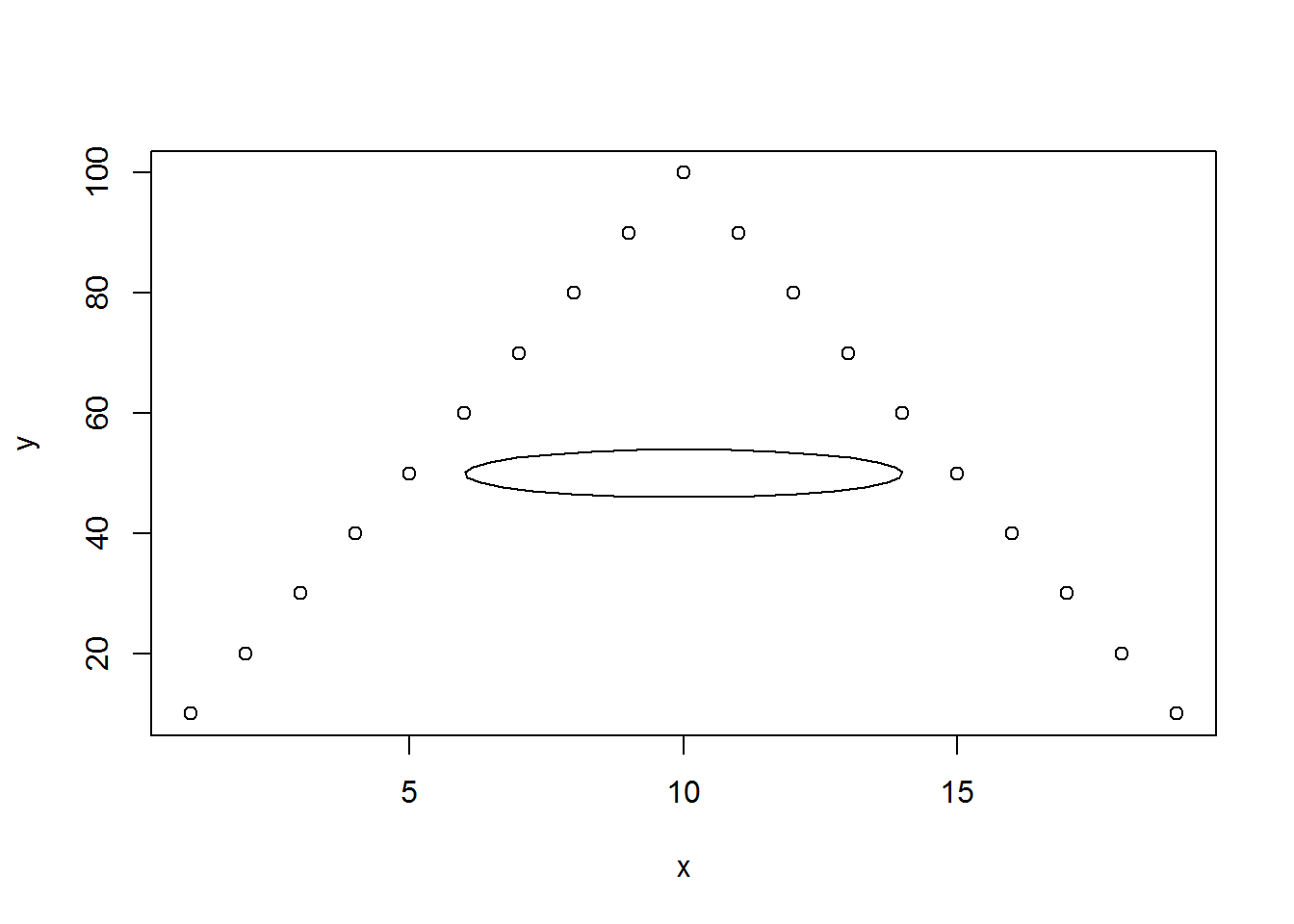Sorry, but this is an ellipse and not a circle. Eight units in the vertical direction is not equal in size to eight units in the horizontal direction.

To make this look like a circle, you need to account for the different user range and the different number of pixels in the vertical direction. If you adjusted just for the different user range (in the above example, adjust by a factor of 90/18=5), you’d get close, but there are two things working against you.

First, the graphics window might not be square. It depends on your defaults.

Second, even if the graphics windw was square, you’d still have an issue because the margins of the graph, by default are not all equal. By default, you have a bit more of a margin at the bottom of the graph than anywhere else.

To get a true circle you need to adjust based on the pixels per unit in the horizontal and vertical dimensions.

``````radians <- seq(0,2*pi,length=30)
plot(x,y)
polygon(true.circle)``````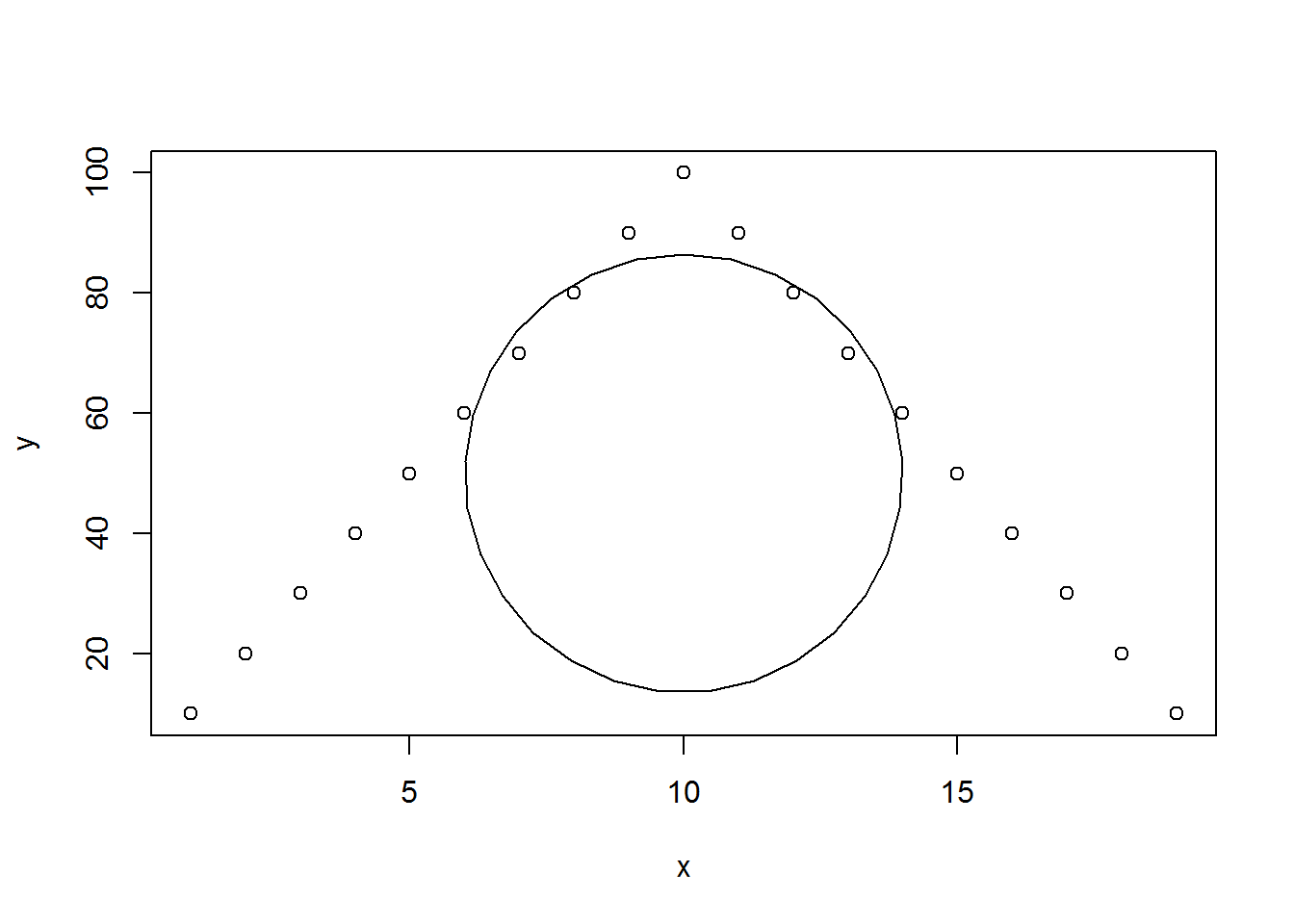Now this looks like a circle. If you resize your graphics window, it will turn into an ellipse again and you have to start afresh.

# Plot margins

You can attack the problem from the outside in by looking not at the size of the plotting region, but instead at the size of the margins.

``````mar.lines <- par("mar")
mar.inches <- par("mai")
mar.lines/mar.inches``````
``##  5 5 5 5``

The “mar” parameter gives you the size of the bottom, left, top, and right margins respectively in terms of “lines.” I’m guessing that a “line” is equal to the height of a single line of normal text.

The “mai” parameter gives you the size of the these margins in inches. On my system, the ratio of “mai” to “mar” indicates that each line is equal to 0.2 inches.

Often you may wish to change the margins of your graph. If, for example, your graph has no axes, no titles, and no labels, then you are wasting a lot of space that would be put to better use in your graph itself.

``````par(mai=rep(0.05,4))
ws.inches <- dev.size()
print(ws.inches)``````
``##  7 5``
``````gs.inches <- par("pin")
print(gs.inches)``````
``##  6.899999 4.899999``

Notice here that the size of the graphing region is almost as big as the size of the entire graphics window.

There are times that you want a perfectly square graph. A perfectly square graph means that the line of unity(y=x) appears as a diagonal line of 45 degrees that goes from the lower left corner to the upper right corner of your graph. To do this you need three (or maybe four) things.

1. open up your graphics window with the same number of inches (and thus the same number of pixels) in the horizontal and vertical directions.
2. set the margins on your graph symmetrically in the horizontal and vertical directions.
3. set up the limits on the x-axis and the y-axis to be identical.
4. You usually don’t need to do this because your default settings will work here, but please make sure that the pixels for inch default settings on your computer are identical in the x direction and the y direction. In some graphics commands, like windows() this is controlled by the xpinch and ypinch parameters.

Since I am using R markdown to create these graphs, I had to insert the parameters fig.width=6 and fig.height=6. This does not display directly in the output from R Markdown. If you are running R directly, you might use the windows(width=6,height=6) command to get the same example started.

``````par(mai=c(1.2,1.2,0.1,0.1))
plot(x,y,xlim=range(x,y),ylim=range(x,y))
abline(a=0,b=1)``````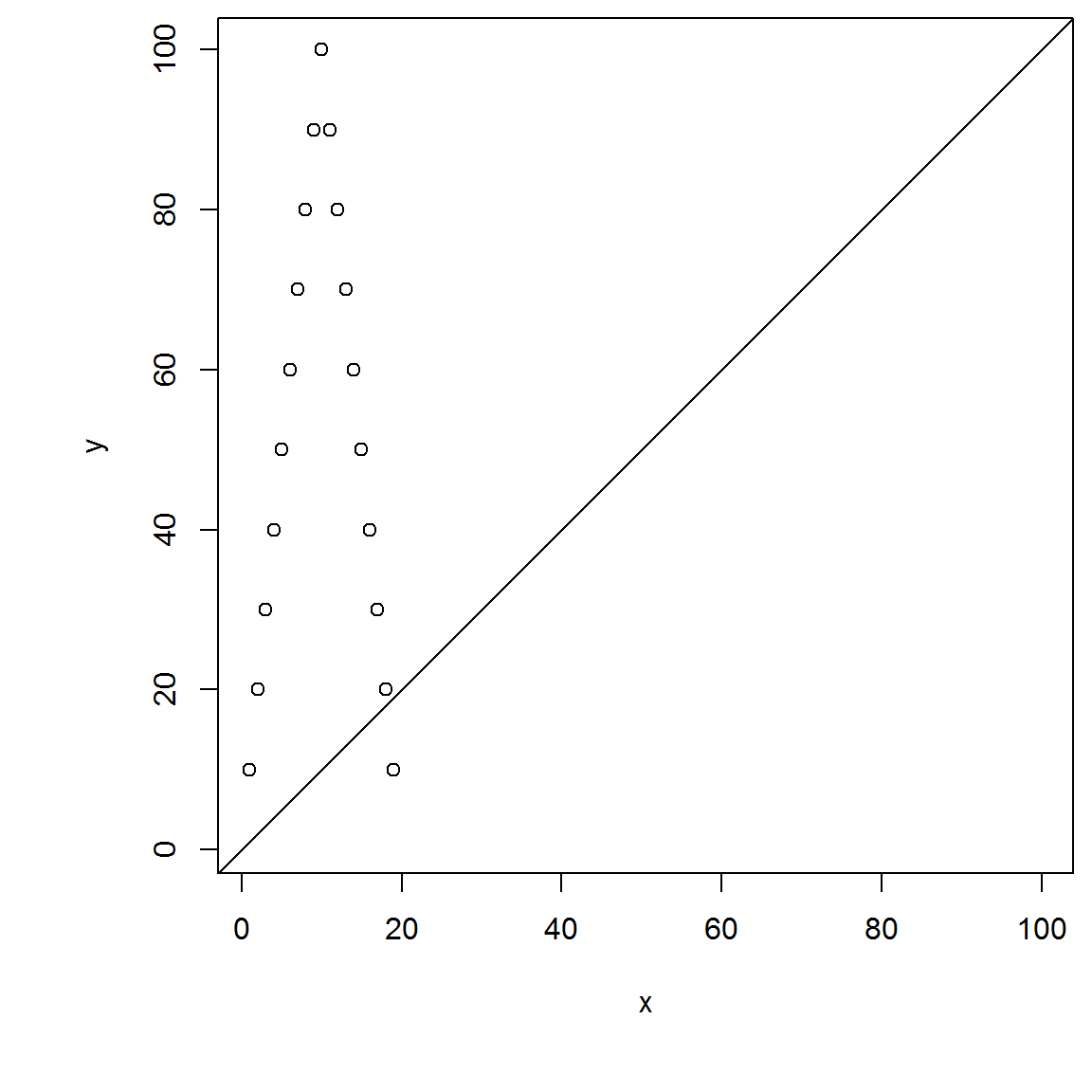## size of text

You should pay attention to how big the text values are in a graph.

``````plot(c(1:10,9:1))
text(10,5,"Hi")
Hi.width.user <- strwidth("Hi",units="user")
print(Hi.width.user)``````
``##  0.5273438``
``````Hi.height.user <- strheight("Hi",units="user")
print(Hi.height.user)``````
``##  0.3844937``
``````x.box <- 10+c(-1,-1,1,1)*Hi.width.user/2
y.box <-  5+c(-1,1,1,-1)*Hi.height.user/2
polygon(x=x.box,y=y.box,border="red")``````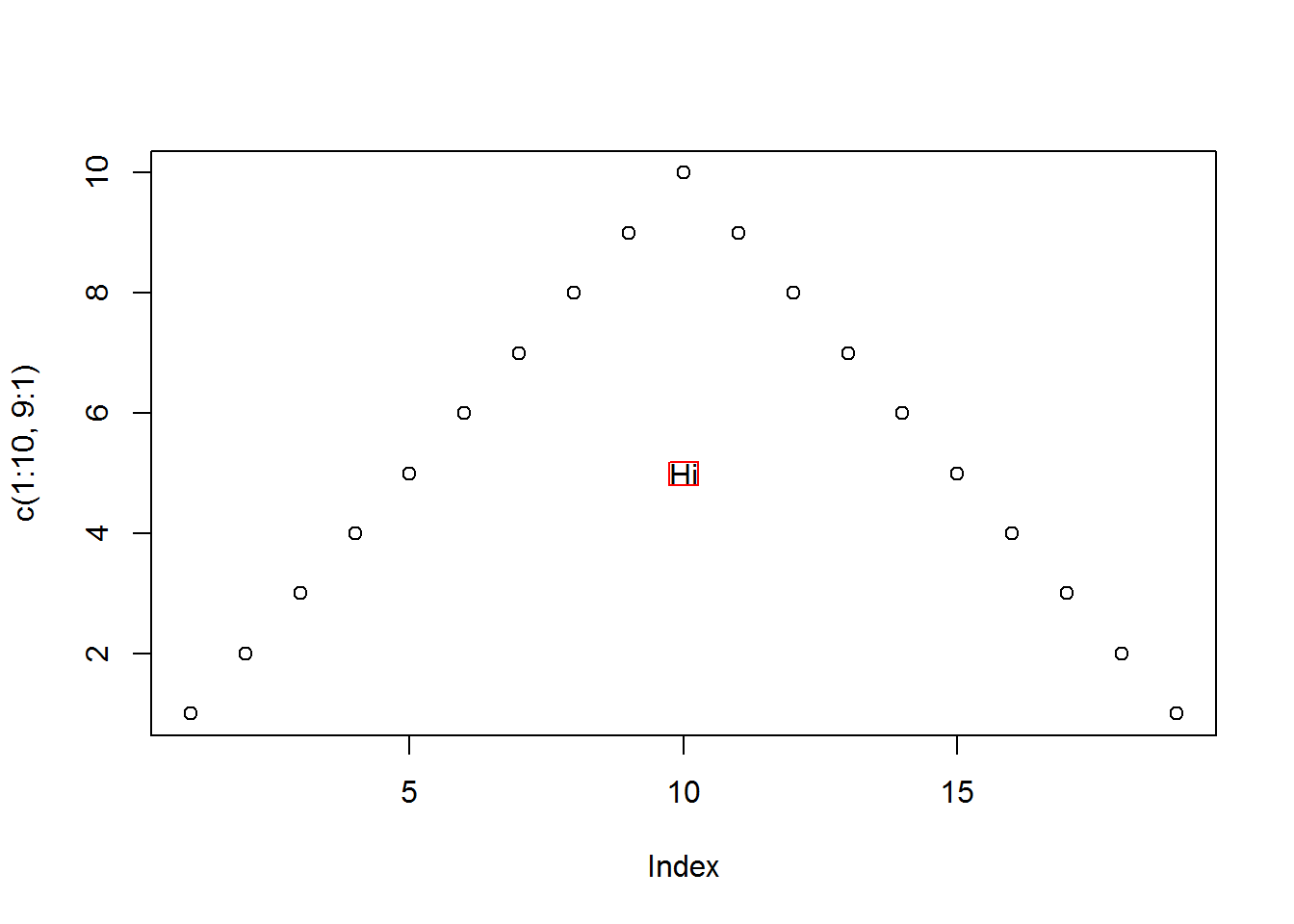``````H.width.user <- strwidth("H")
print(H.width.user)``````
``##  0.4042969``
``````i.width.user <- strwidth("i")
print(i.width.user)``````
``##  0.1230469``
``````Hi.width.inches <- strwidth("Hi",units="inches")
print(Hi.width.inches)``````
``##  0.15625``
``````Hi.width.figure <- strwidth("Hi",units="figure")
print(Hi.width.figure)``````
``##  0.02232143``
``Hi.width.figure*dev.size(units="in")``
``##  0.15625``

The strwidth and strheight functions will give you exact sizes of a text string. You can control the measurement scale with the units parameter. Notice that the width of the string “Hi” (0.527) is equal to the width of the string “H” (0.404) plus the width of the string “i” (0.123)

The units=“figure” parameter will give you the width or height of a string as a proportion of the window size. Multiplying this proportion by the size of the window in inches gives you the size of the string in inches as shown above.

There are several parameters in par (cin, cra, cxy) that also provide you with information about the size of characters, but for what they call a “default character.” This gives you a rough approximation to the size of a string and may be useful for settings where the exact string might vary.

## Size of graphs when multiple graphs appear in the same window

You can have multiple graphs in the same window, using the mfrow or mfcol parameters in par or using the layout function. When you have multiple graphs, the value produced by the fin parammeter of the par function changes.

``````par(mfrow=c(2,2))
plot(x,y)
plot(x,110-y)
plot(y,x)
plot(110-y,x)``````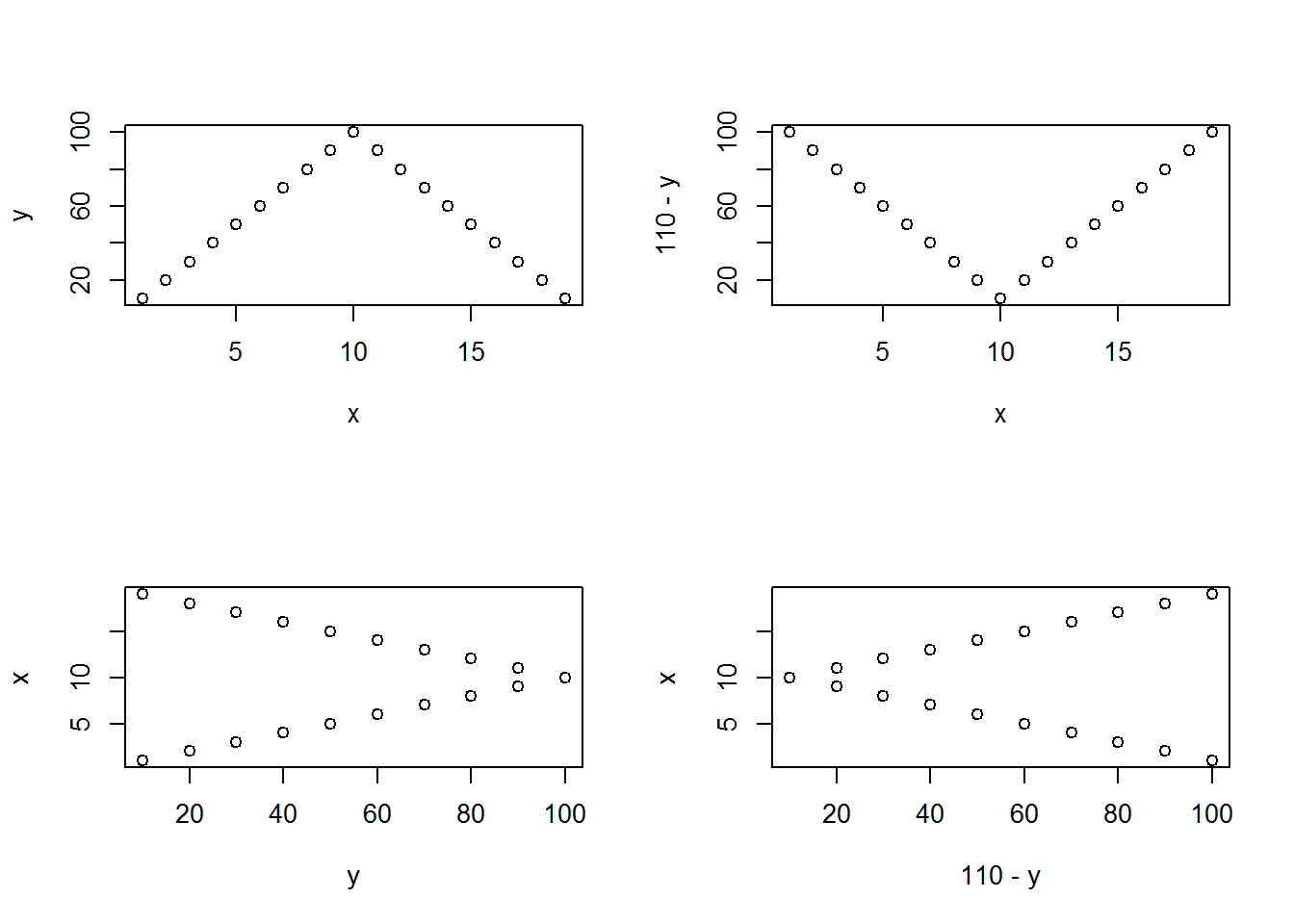``````fig.size.inches <- par("fin")
print(fig.size.inches)``````
``##  3.5 2.5``

Notice that the size of the figure is half the size of the graphics window.

When you have multiple graphs in a single window, you can define an “outer” margin that surrounds all of the graphs. This allows you, for example, to put a super title across the top. To experiment with this, look at the oma, omd, and omi parameters of the par function and the mtext function.

There’s a lot more to this, but I hope this helps you get started.

## Save results for later use

``save.image("plotsize.RData")``